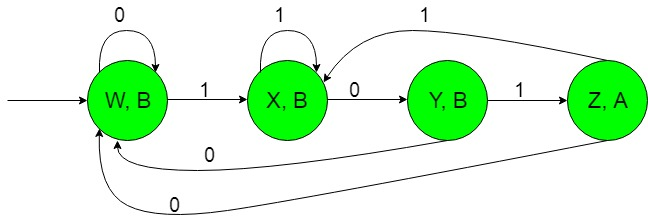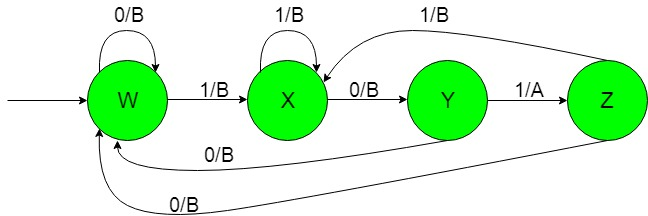# Finite Automata with Output (Set 6)

• Last Updated : 20 Nov, 2019

Prerequisite: Mealy and Moore Machines, Difference between Mealy machine and Moore machine
In this article, we will see some designing of Finite Automata with Output, i.e., Moore and Mealy machines.

Problem: Construction of the machines that take set of all string over {0, 1} as input and produce ‘A’ as output if the input contains ‘101’ as the substring or the input string starts with ‘101’ or ends with ‘101’.
That is here we have,
Ε = {0, 1} and
Δ = {A, B}
where Ε and Δ are the input and output alphabet respectively.

Attention reader! Don’t stop learning now.  Practice GATE exam well before the actual exam with the subject-wise and overall quizzes available in GATE Test Series Course.

Learn all GATE CS concepts with Free Live Classes on our youtube channel.

The required Moore machine is constructed below:-Explanation:
In the above diagram, the initial state ‘w’ on getting ‘0’ as the input it remains in the state of itself and prints ‘B’ as the output and on getting ‘1’ as the input it transits to a state ‘X’ and prints ‘B’ as the output. The state ‘X’ on getting ‘1’ as the input it remains in the state of itself and prints ‘B’ as the output and on getting ‘0’ as the input it transmits to the state ‘Y’ and prints ‘B’ as the output and so on for the remaining states.
Thus finally above Moore machine can easily give ‘A’ as the output on getting ‘101’ as input substring.

The required Mealy machine is constructed below:-Explanation:
In the above diagram, the initial state ‘w’ on getting ‘0’ as the input it remains in the state of itself and prints ‘B’ as the output and on getting ‘1’ as the input it transits to a state ‘X’ and prints ‘B’ as the output. The state ‘X’ on getting ‘1’ as the input it remains in the state of itself and prints ‘B’ as the output and on getting ‘0’ as the input it transmits to the state ‘Y’ and prints ‘B’ as the output and so on for the remaining states.
Thus finally above Moore machine can easily give ‘A’ as the output on getting ‘101’ as input substring.

My Personal Notes arrow_drop_up